• 累计撰写 164 篇文章
• 累计创建 25 个标签
• 累计收到 208 条评论

### 目 录CONTENT# 排序算法:归并排序的理解与实现2020-04-04 / 0 评论 / 1 点赞 / 262 阅读 / 3,278 字

## 归并图解示例

• 如图所示，我们先将1号数组和2号数组的内容放进3号数组里• 如图所示，我么将数组拆分为两个小数组，leftright，用i指向left数组的0号元素，用j指向right数组的0号元素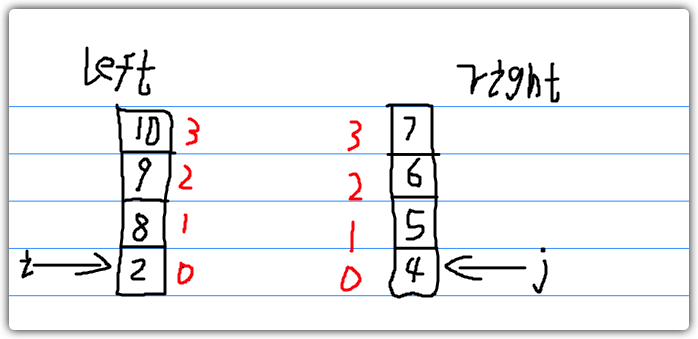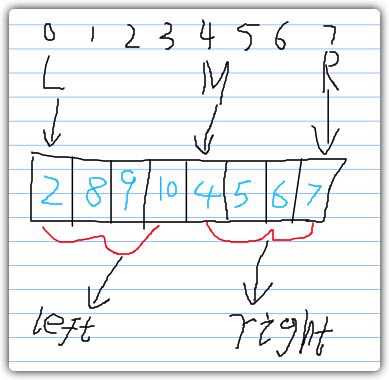## 归并排序图解示例• 将序列对半分割（2段）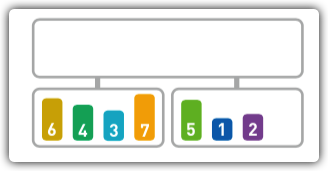• 在继续往下分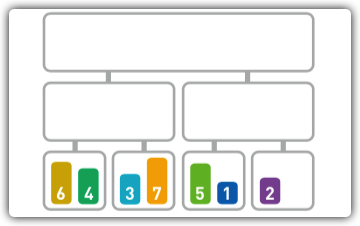• 分割完毕，结下来对分割后的元素进行合并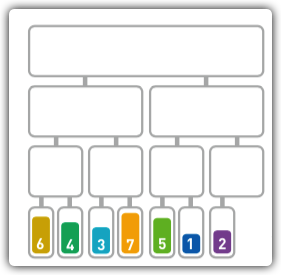• 将6与4进行合并，合并后的顺序为[4,6]• 接下来把3和7进行合并，合并后的顺序为[3,7]• 此时，我们产生了两组从小到大排列的数据，符合了归并的要求，我们将这两组数据代归并中，进行合并。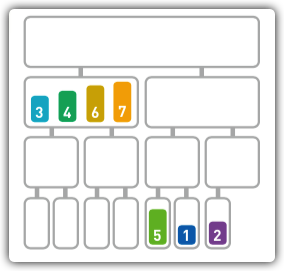• 此时，我们发现还剩余两组数据未进行排序，我们递归上述操作，将这两组数据进行合并• 合并完后，我们发现又剩余两组数据，符合了归并的要求，我们继续调用归并，将这两组数据进行合并，排序完成。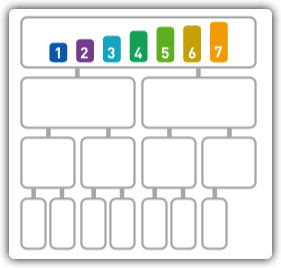## 用JS实现归并排序

### 归并的实现

• 声明归并函数：

• 参数arr为两组从小到大排序的数组，将其合并成一个以后的数组。
• 参数L为数组的起点索引
• 参数M为数组的中间点(分割点),用于标识两组从小到大排序的数组，M左边的数据为一个数组(leftArr)，M本身以及它右边的数据为一个数组(rightArr)。
• 参数R为数组的终点索引
• 分别计算左、右数组的长度

• 左边数组的长度为M - L
• 右边数组的长度为R - M + 1
• 声明左、右数组，初始化其长度

• 根据中间值，分别将arr中的数据填充到左、右数组

• 左数组: 从L填充到M(不包含M)
• 右数组: 从M(包含M)填充到R
• 将两组数据进行合并(从小到大进行排序)

• 声明3个变量：i, j, k

• i指向左侧数组的每一项
• j指向右侧数组的每一项
• k指向合并后的数组的每一项
• 将左侧数组的每一项数据与右侧数组的每一项数据进行大小比较

• 当前遍历到左侧数组的数据 < 当前遍历到的右侧数组的数据，则arr的k项为当前左侧数组的数据。i自增，k自增。
• 当前遍历到左侧数组的数据 > 当前遍历到的右侧数组的数据，则arr的k项为当前右侧数组的数据。j自增，k自增。
• 判断左、右数组是否比较完成

• 如果左侧数组的数据已经比较完，右侧数组的数据还未比较完，则arr的k项就为右侧数组的剩余项。
• 如果右侧数组的数据已经比较完，左侧数组的数据还未比较完，则arr的k项就为左侧数组的剩余项。

/**
* 归并函数
* @param arr
* @param L 数组的起点
* @param M 数组的分割点
* @param R 数组的终点
*/
const merger = function (arr, L, M, R) {
// 左边数组大小和右边数组大小
let left_size = M - L;
let right_size = R - M + 1;
// 声明左边数组和右边数组
let leftArr = new Array(left_size);
let rightArr = new Array(right_size);
let i,j,k;

// 填充左数组(从L开始到M结束)
for (i = L; i < M; i++){
leftArr[i-L] = arr[i];
}
// 填充右数组(从M开始到R结束)
for (i = M; i <= R; i++){
rightArr[i-M] = arr[i];
}

// 数组合并
i = 0; j = 0; k = L;
while (i < left_size && j < right_size){
// 如果左边数组的i项小于右边数组的j项，则数组的k项就为左边数组的i项。否则数组的k项就为右边数组的j项
if(leftArr[i] < rightArr[j]){
arr[k] = leftArr[i];
i++;
k++;
}else{
arr[k] = rightArr[j];
j++;
k++;
}
}
// 当右边数组到顶部后，左边数组还未到顶部，则将剩余元素放进arr中
while (i < left_size){
arr[k] = leftArr[i];
i++;
k++
}
// 当左边数组到顶部后，右边数组还未到顶部，则将剩余元素放进arr中
while (j < right_size){
arr[k] = rightArr[j];
j++;
k++;
}
};


• 测试下我们写的归并函数
const dataArr = [2,8,9,10,4,5,6,7];
/*测试合并*/
merger(dataArr,0,4,7);
// 合并后的数据
console.log(dataArr);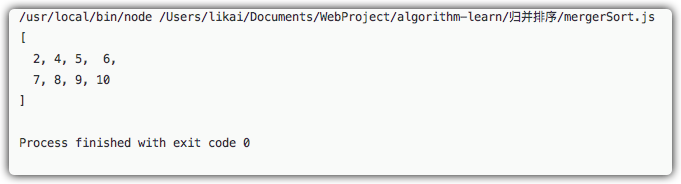### 归并排序的实现

• 计算中间值: (L + R) / 2
• 分割左、右数组
• 合并分割后的数据
• 递归操作(直至L = R)

const mergerSort = function (arr,L,R) {
if(L===R){
// 数据已经切割完毕
return false;
}
else{
// 计算中间值(向下取整)
let M = Math.floor((L + R) / 2);
// 分割后,把左边的数据进行一次归并排序
mergerSort(arr,L,M);
// 对右边的数据进行一次归并排序
mergerSort(arr,M+1,R);
// 合并两边的数据
merger(arr,L,M+1,R)
}
};

• 测试下我们写的归并排序
const dataArr = [6,4,3,7,5,1,2];
/*测试排序*/
mergerSort(dataArr,0,dataArr.length - 1);
// 合并后的数据
console.log(dataArr);## 写在最后

• 文中使用的图片源自《我的第一本算法书》，如若侵权，请评论区留言，作者立即删除相关图片。
• 文中如有错误，欢迎在评论区指正，如果这篇文章帮到了你，欢迎点赞和关注😊
• 本文首发于掘金，未经许可禁止转载💌
1